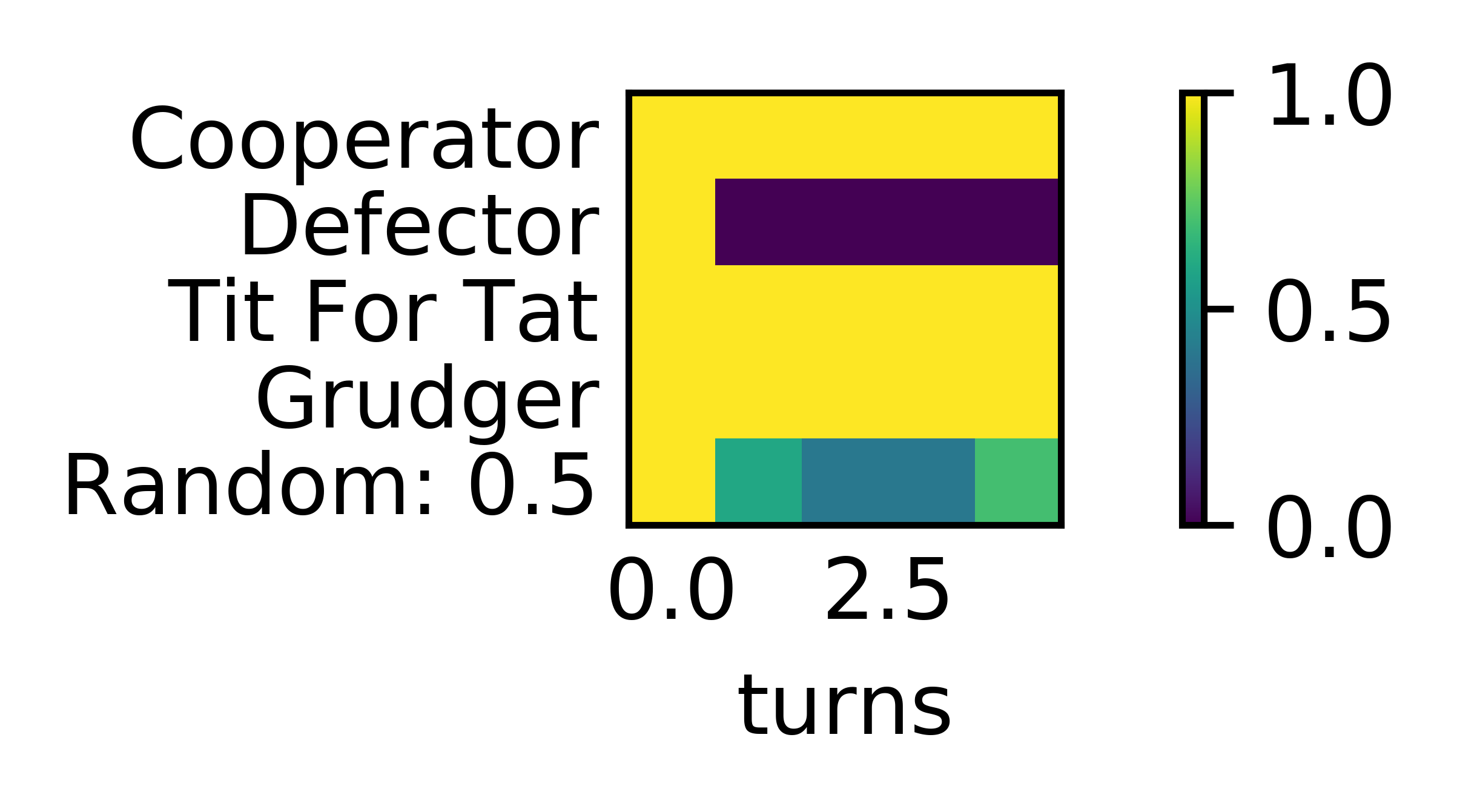# Fingerprinting¶

## Ashlock Fingerprints¶

In [Ashlock2008], [Ashlock2009] a methodology for obtaining visual representation of a strategy’s behaviour is described. The basic method is to play the strategy against a probe strategy with varying noise parameters. These noise parameters are implemented through the JossAnnTransformer. The Joss-Ann of a strategy is a new strategy which has a probability x of cooperating, a probability y of defecting, and otherwise uses the response appropriate to the original strategy. We can then plot the expected score of the strategy against x and y and obtain a heat plot over the unit square. When x + y >= 1 the JossAnn is created with parameters (1-y, 1-x) and plays against the Dual of the probe instead. A full definition and explanation is given in [Ashlock2008], [Ashlock2009].

Here is how to create a fingerprint of WinStayLoseShift using TitForTat as a probe:

>>> import axelrod as axl
>>> axl.seed(0)  # Fingerprinting is a random process
>>> strategy = axl.WinStayLoseShift
>>> probe = axl.TitForTat
>>> af = axl.AshlockFingerprint(strategy, probe)
>>> data = af.fingerprint(turns=10, repetitions=2, step=0.2)
>>> data
{...
>>> data[(0, 0)]
3.0


The fingerprint method returns a dictionary mapping coordinates of the form (x, y) to the mean score for the corresponding interactions. We can then plot the above to get:

>>> p = af.plot()
>>> p.show()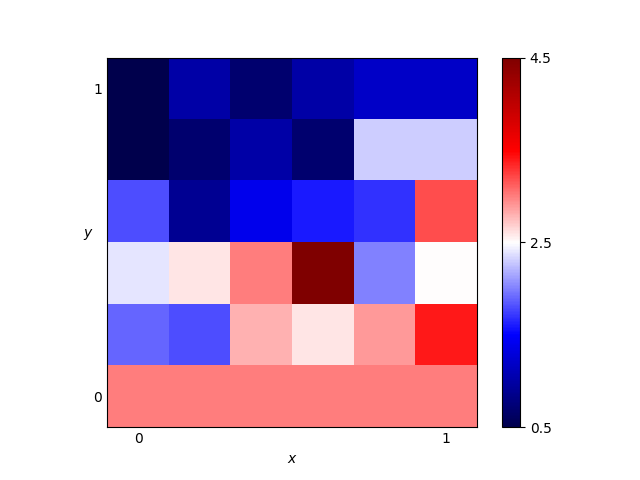In reality we would need much more detail to make this plot useful.

Running the above with the following parameters:

>>> af.fingerprint(turns=50, repetitions=2, step=0.01)


We get the plot: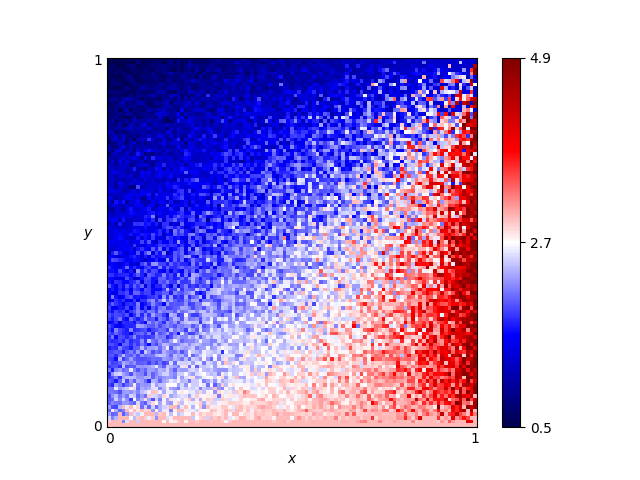We are also able to specify a matplotlib colour map, interpolation and can remove the colorbar and axis labels:

>>> p = af.plot(cmap='PuOr', interpolation='bicubic', colorbar=False, labels=False)
>>> p.show()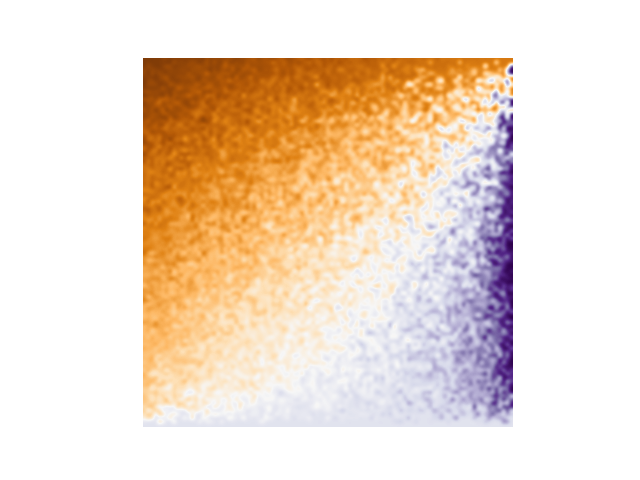Note that it is also possible to pass a player instance to be fingerprinted and/or as a probe. This allows for the fingerprinting of parametrized strategies:

>>> axl.seed(0)
>>> player = axl.Random(p=.1)
>>> probe = axl.GTFT(p=.9)
>>> af = axl.AshlockFingerprint(player, probe)
>>> data = af.fingerprint(turns=10, repetitions=2, step=0.2)
>>> data
{...
>>> data[(0, 0)]
4.4...


## Transitive Fingerprint¶

Another implemented fingerprint is the transitive fingerprint. The transitive fingerprint represents the cooperation rate of a strategy against a set of opponents over a number of turns.

By default the set of opponents consists of 50 Random players that cooperate with increasing probability. This is how to obtain the transitive fingerprint for TitForTat:

>>> axl.seed(0)
>>> player = axl.TitForTat()
>>> tf = axl.TransitiveFingerprint(player)
>>> data = tf.fingerprint(turns=40)


The data produced is a numpy array showing the cooperation rate against a given opponent (row) in a given turn (column):

>>> data.shape
(50, 40)


It is also possible to visualise the fingerprint:

>>> p = tf.plot()
>>> p.show()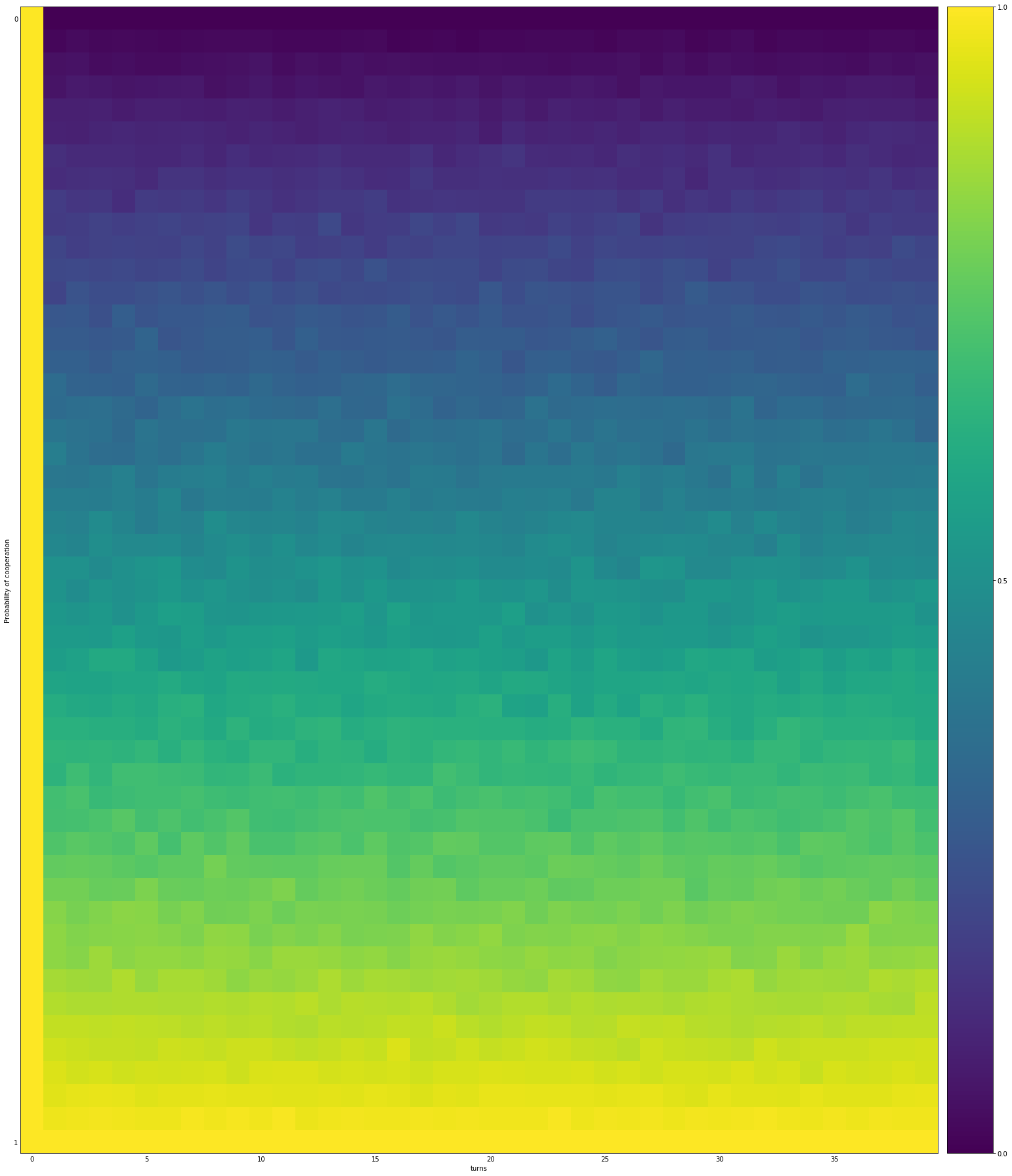It is also possible to fingerprint against a given set of opponents:

>>> axl.seed(1)
>>> opponents = [s() for s in axl.demo_strategies]
>>> tf = axl.TransitiveFingerprint(player, opponents=opponents)
>>> data = tf.fingerprint(turns=5, repetitions=10)


The name of the opponents can be displayed in the plot:

>>> p = tf.plot(display_names=True)
>>> p.show()